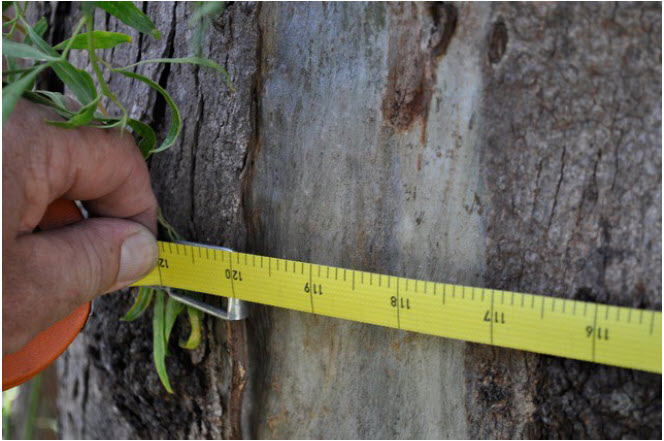Go to website

# reSolve: Circumference

This sequence of three lessons explores the properties of circles, in particular the relationship between the diameter and circumference. In the first lesson, students use a cylinder containing three tennis balls to understand that a circumference is 'three and a bit' times the diameter. They then complete five different activities measuring circular objects to determine a more accurate value for pi (π). Finally, students are given a range of investigation activities to consolidate their learning. Each lesson is outlined in detail including curriculum links, vocabulary, supporting PowerPoint presentations, materials needed, sample answers, discussion points and student resources. This sequence is part of the reSolve: Mathematics by Inquiry program.

Year level(s) Year 8
Audience Teacher
Purpose Teaching resource
Teaching strategies and pedagogical approaches Mathematics investigation

## Curriculum alignment

Curriculum connections Numeracy
Strand and focus Geometry, Build understanding, Apply understanding
Topics Shapes and objects, Angles and geometric reasoning
AC: Mathematics content descriptions
ACMMG197 Investigate the relationship between features of circles such as circumference, area, radius and diameter. Use formulas to solve problems involving circumference and area
National numeracy learning progression Understanding geometric properties - UGP6